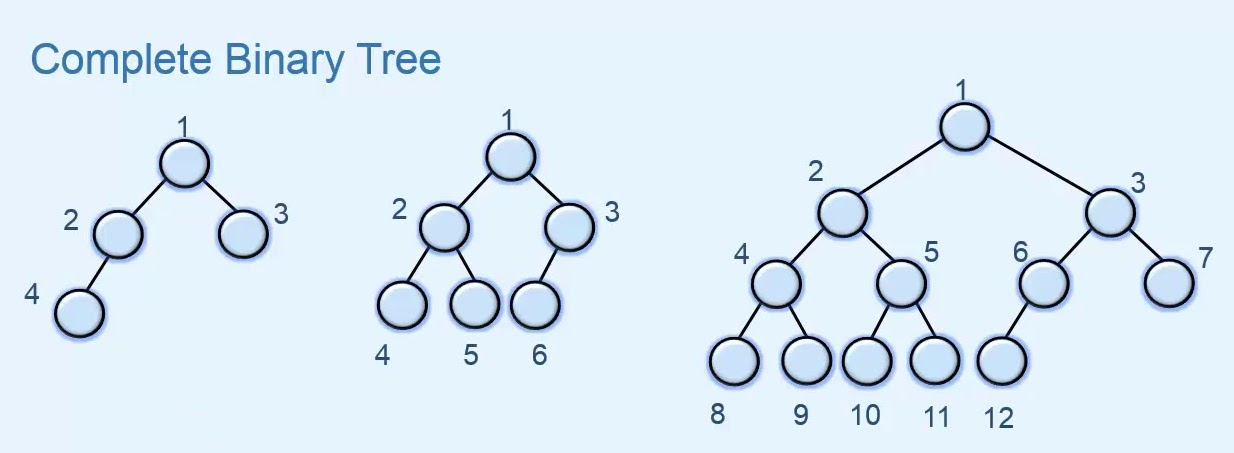#### Why do we even need the Heap data structure ?

We need Heap when our solution for a problem demands to use a data structure that has the following characteristics:
• Efficiently find the smallest (or largest) element in a set.
• The cost to find the smallest (largest) element takes constant time.

• The cost to delete the smallest (largest) element takes time proportional to the log of the number of elements in the set.
• The cost to add a new element takes time proportional to the log of the number of elements in the set.

#### What are some of the interesting characteristics of Heaps that sets it apart from other data structures ?

• Heap is a data structure used to efficiently find the smallest (or largest) element in a set.
• Min-heaps make it easy to find the smallest element. Max-heaps make it easy to find the largest element.
• Heaps are based upon Complete Binary Trees. These trees maintain the heap property.
A complete binary tree is a binary tree in which every level, except possibly the last, is completely filled, and all nodes are as far left as possible.• The Heap invariant:
1. For a Min Heap the value of the every parent node is lower than values of both of its child nodes.
2. For a Max Heap the value of the every parent node is greater than values of both of its child nodes.
• The trees must be mostly balanced for the costs listed below to hold.
• Access to elements of a heap usually have the following costs.
1. The cost to find the smallest (largest) element takes constant time.
2. The cost to delete the smallest (largest) element takes time proportional to the log of the number of elements in the set.
3. The cost to add a new element takes time proportional to the log of the number of elements in the set.
• Heaps can be implemented using arrays (using the tree embedding described later in this chapter) or by using balanced binary trees like leftist trees
• Heaps form the basis for an efficient sort called Heap Sort that has cost proportional to n*log(n) where n is the number of elements to be sorted.
• Heaps are the data structure most often used to implement priority queues.

#### Array embedded Binary Trees:

Heaps are nothing but Complete Binary Trees. So we need to know how we could implement binary trees using arrays.

Binary trees can be efficiently stored in arrays by using an encoding that stores tree elements at particular indexes in the array. One can compute the indexes of that tree nodes left-child, right-child, and parent node using a simple formula.
We can envision this process by the following picture:Several invariants must hold for this to work.
• The array must be larger than the number of nodes in the tree.
• The tree must be balanced. Either a full-tree or a complete-tree.
• The array must be zero indexed (the first slot must have index zero) or the formulae won't work out.
• An extra variable indicating the last slot in the array that is in use. This index notes the rightmost node in the last rank of the tree. This would be index 9 in the picture above, indicating the node labelled E.

The formulae for computing the tree child and parent relationships in the array are:
• index of root in zero-based indexed array = 0
• index left_child of node with index i in array = (i * 2) + 1
• index of right_child of node with index i in array = (i * 2) + 2
• index of parent of child node j = (j - 1) / 2

Please go through the below two chapters where we will be digging deeper into the four most important Heap Operations:

#### Instructor: